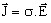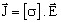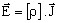# Electric behavior of materials: definitions

## Definition

The macroscopic dependence linking the values of the electric current density J and the electric field strength E in any point of material is called the law of material electric behavior.

This lawrepresents the local formulation of Ohm's law.

## Notation

This law is written in the form:orwhere:

• σ is the conductivity tensor
• ρ is the resistivity tensor

The electric conductivity σ is a measure of how well a material allows electric current flow. ( It stands for the capacity of the material that contains movable electric charges to make them flow under the action of the electric field.)

The electric resistivity ρ is a measure of how strongly a material opposes the current flowing.